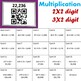# Basic Operations QR Code Scavenger Hunt 4 Pack BUNDLESubject
Resource Type
File Type

PDF

(2 MB|40 pages)
Standards
• Product Description
• StandardsNEW

Do your students need a little more practice with multi-digit addition, subtraction, multiplication and division? These fun, QR code scavenger hunts are just what you need to give them a little extra practice in a fun and engaging way.

These 4 scavenger hunts will get your students up and moving around the room using technology to practice solving three digit addition, three digit subtraction, two and three digit multiplication and division with up to 4 digit dividends and 2 digit divisors.

These scavenger hunts use QR codes which are very engaging to students! This activity requires students to use a mobile device with a QR code reader such as a tablet, iPad, iPhone, etc.

Students scan the start card to get the first problem and then look for the card with the answer they got to scan for the next problem.

This bundle includes 4 complete QR code scavenger hunts. Each set includes 20 scavenger hunt task cards, 1 or 2 options for student record sheets and a full answer key.

These 4 products are also available as single purchases

Three Digit Addition QR Code Scavenger Hunt

Three Digit Subtraction QR Code Scavenger Hunt

Double and Triple Digit Multiplication QR Code Scavenger Hunt

Long Division QR Code Scavenger Hunt

To learn more about how I use QR code scavenger hunts in my own classroom, check out my blog post.

To learn more about how I find creative ways for kids to practice math, check out my blog. You can also find me on Facebook and Pinterest.

Be the first to know about my new products, freebies and sales! Look for the green star near the top right of the page and click it to become a follower.

Fluently divide multi-digit numbers using the standard algorithm.
Find whole-number quotients of whole numbers with up to four-digit dividends and two-digit divisors, using strategies based on place value, the properties of operations, and/or the relationship between multiplication and division. Illustrate and explain the calculation by using equations, rectangular arrays, and/or area models.
Fluently multiply multi-digit whole numbers using the standard algorithm.
Find whole-number quotients and remainders with up to four-digit dividends and one-digit divisors, using strategies based on place value, the properties of operations, and/or the relationship between multiplication and division. Illustrate and explain the calculation by using equations, rectangular arrays, and/or area models.
Multiply a whole number of up to four digits by a one-digit whole number, and multiply two two-digit numbers, using strategies based on place value and the properties of operations. Illustrate and explain the calculation by using equations, rectangular arrays, and/or area models.
Total Pages
40 pages
Included
Teaching Duration
1 Week
Report this Resource to TpT
Reported resources will be reviewed by our team. Report this resource to let us know if this resource violates TpT’s content guidelines.\$9.75
List Price:
\$17.00
You Save:
\$7.25